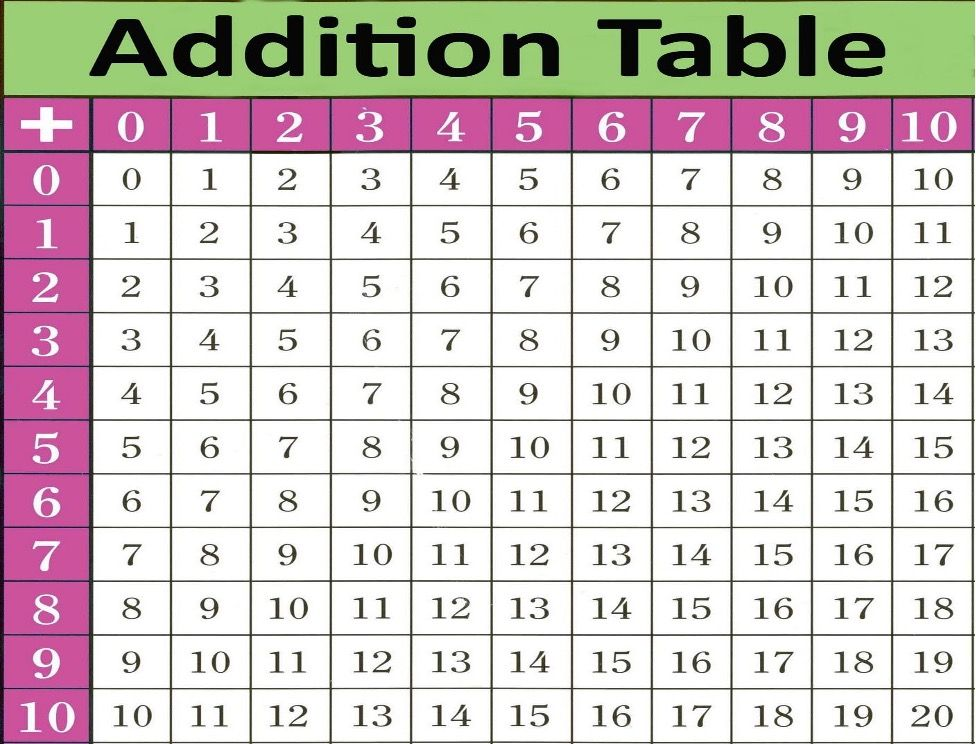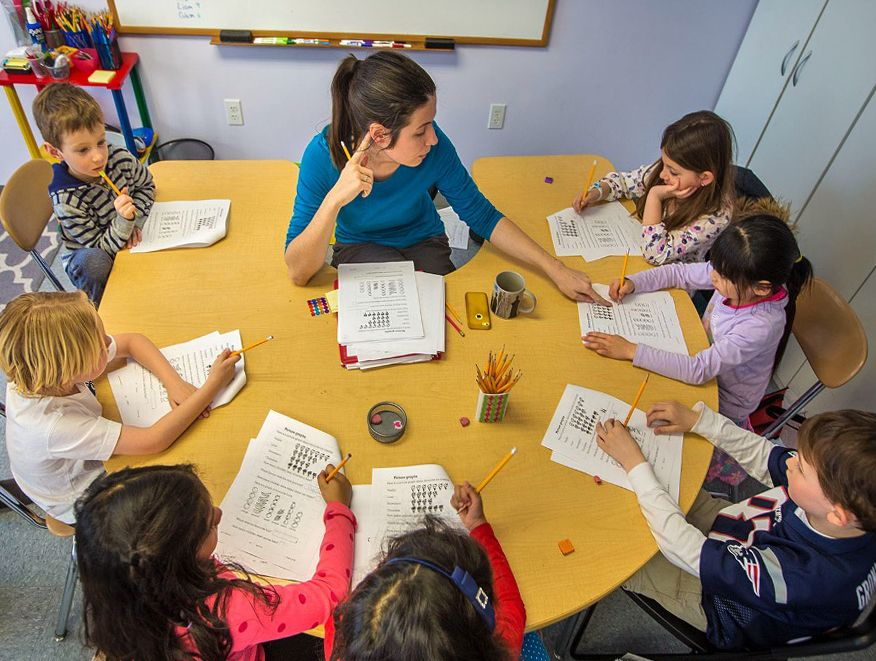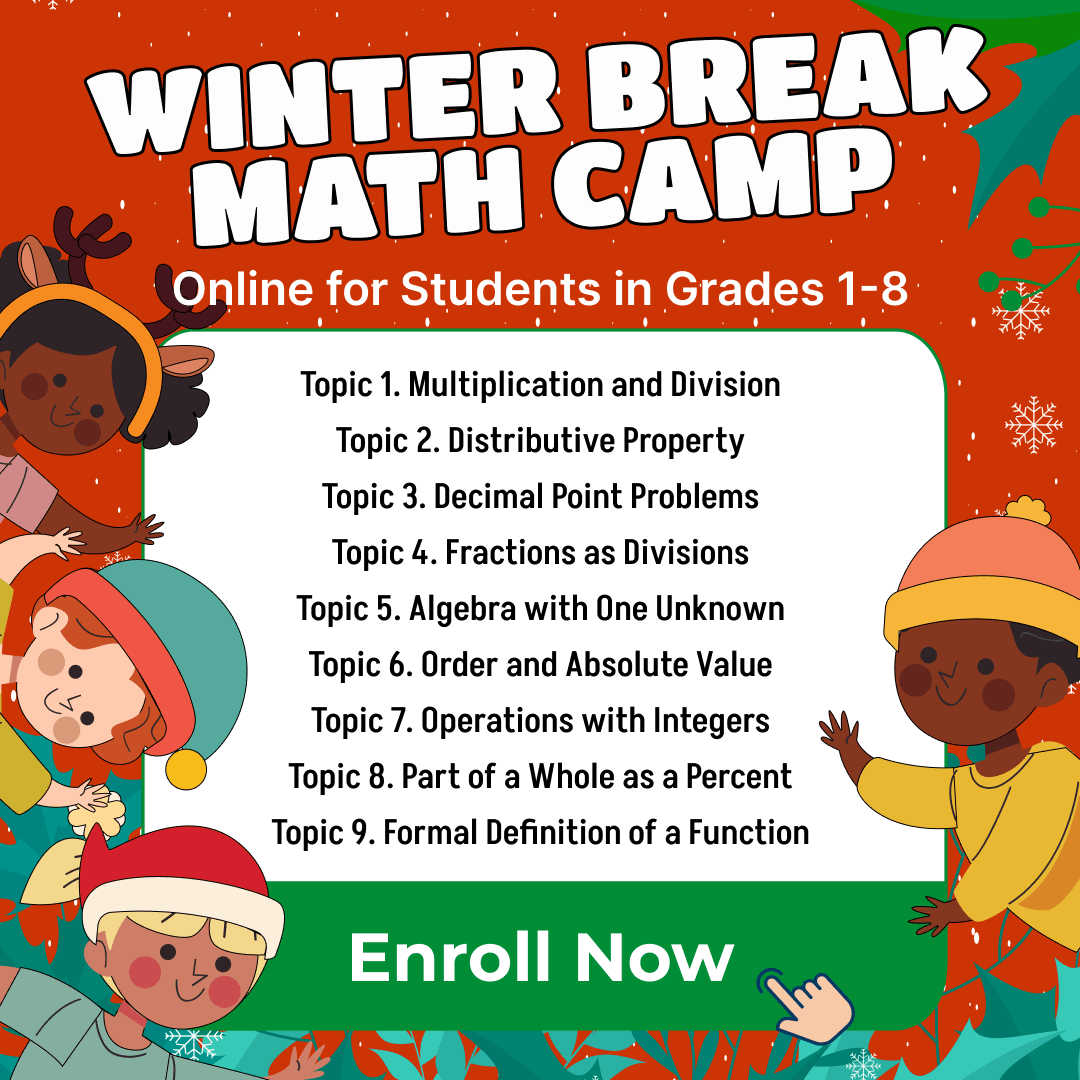TeachingDo you want to ease your kids’ way through math step by step? Indeed, when it comes to numbers, addition is one of the first mathematical skills most students learn. In its simplest form, we equate addition to counting objects. This article will acquaint you with the most important ideas about addition facts.

Addition is one of the four basic operations of arithmetic, with others being subtraction, multiplication, and division. We denote addition by the plus sign (+) and use it to find out the total number of objects in a set. For instance, the addition of two whole numbers is the total amount of those values combined.

Addition is not just a process of adding numbers. It is also a foundation for numerous math operations, ranging from simple concepts like “two plus two equals four” to more complex topics like algebraic expressions.

For example, we can express the sum of two numbers using their symbols as follows:

7 + 9 = 16

In this case, 7 + 9 means that we have to add 7 and 9 together and get the answer 16.  We have to put the values of 7 and 9 next to each other on a number line and then count 16 steps from zero.One can perform addition facts with more than two numbers at a time. This way, you can find out how many objects there are in a set if all you know is how many of them there are in each group. For example, if you have 2 apples, 4 oranges, and 6 pears, you could determine how many fruits you have by adding 2 + 4 + 6 = 12 fruits.

## What are addition facts to 10?

Addition facts to 10 refer to all number pairs you can make using numbers from 0 to 9 that add up to 10 or less.

Also, addition facts to 10 are sums of numbers less than or equal to 10. For example, 3 + 4 = 7 is one of the addition facts to 10. It involves two numbers less than or equal to 10. With 3 plus 4, the sum is 7, which is also less than or equal to 10.

Math for Kids

Is Your Child Struggling With Math?
1:1 Online Math TutoringStudents need to memorize addition math facts to 10 as quickly as possible. These facts are the addition facts that stick to the child’s memory and are easy to learn.

One can practice addition facts to 10 using flashcards, a worksheet, or playing a game. The ability to recall basic addition facts quickly and accurately is a fundamental skill essential for success in mathematics.

The primary goal of learning these addition facts is to develop fluency in solving math problems. A child who knows addition facts can quickly recall that 6 + 4 = 10 without having to count on their fingers or use other strategies. Children can also perform subtraction problems using these same facts, such as 10 – 4 = 6.

The biggest advantage of learning addition facts to 10 is that kids have them at their fingertips. It can be especially important if a child will be taking higher-level math courses in the future. If kids know basic math facts, they do not have to waste time looking up solutions in a book or with a calculator.

The addition facts table is a great visual support for studying at home or in class.## What are addition facts to 20?

Addition facts to 20 are facts that use numbers from 0 to 20 the addition of which results in a sum of 20 or less. For example, 10 + 10 is an addition fact to 20, so is 0 + 2. Knowledge of addition facts to 20 is vital for students in first and second grades because it helps learn mental math techniques.

Addition facts to 20 are essential for fluency in mathematics. Students should memorize them not to calculate simple problems each time they need to use one. Kids can then work on more complex math problems such as multi-digit addition without worrying about the individual sums within those equations.Addition doubles facts are additions of two similar digits of any existing number below 20. You should teach your child to add these numbers before learning the bigger ones. The easiest way to remember these addition facts is by using a mnemonic device, which is an easy way of remembering something new through a phrase or story.

Also, you can use addition facts games as a fun way to help kids learn. You can apply these games at home or in the classroom. Some of these games require you to download a free app and play them on your computer.

## Conclusion

Now you know how younger children can learn addition facts up to 20, what strategies are the most effective for learning addition facts, and how adults can help kids improve their math skills. Remember that only practice makes perfect and it is the only way for learning and improving a child’s math skills.

Winter Break Math Camp• Boost Math Skills this Winter Break at our Camp, Perfect for Students in Grades 1-8!

Winter Break Math Camp
Enhance Math Skills with Our Program, Perfect for 1st-8th Graders, Aligned with School Curriculum!